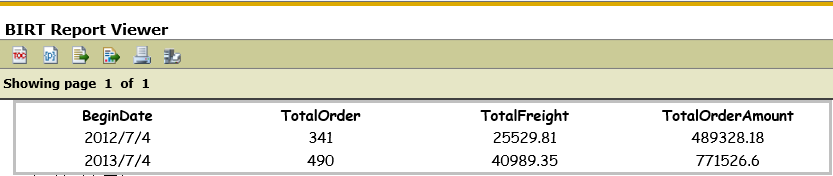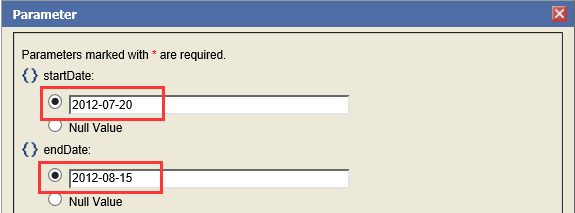## Wednesday, June 19, 2019

### Dynamic Grouping of crosstab in BIRT

Source：
https://forums.opentext.com/forums/discussion/173783/dynamic-grouping-of-crosstab-in-birt#latest

In business filling-in, sometimes it is necessary to make dynamic grouping of crosstab statistics based on time interval, and realize dynamic grouping by day, week, month and year according to the length of time interval. For example, according to business needs, an enterprise needs to achieve order statistics in a period of time according to two parameters (start date, end date). The statistical rules are as follows:

Example: Calculate the difference between dates based on the input parameter values.
Var diff = end date - start date                 ----- days apart
If（diff <15）
Grouping “date group” to display dates
If（diff>15）
Grouping “date group” to display weeks
If（diff >30）
Grouping “date group” to display months
If（diff>365）
Grouping “date group” to display years

Are the little buddies a little overwhelmed? The above problem is essentially a problem of data preparation, but the way of code in SQL or scripted data sources is hard to write and heavy workload; the way of hiding columns in reports is neither universal nor straightforward. A better solution, then, is to introduce esProc into the reporting tool, which will make it easy to solve all kinds of problems. Next, we will take Birt Reporting Tool as an example to introduce the implementation process. For other reporting tools, they are similar.

In this case, according to the input parameters, the total number of orders, freight charges and order amounts of enterprises from 2012-07-04 to 2014-05-06 are counted. The original data in the table "ORDERS" is as follows:Let's look directly at esProc's SPL code to solve this problem:
 A B C 1 =connect("demo") // Connect the database 2 =A1.query("select   ORDERID,ORDERDATE,FREIGHT,ORDERAMOUNT from ORDERS where ORDERDATE >=? and   ORDERDATE <=? ",startDate,endDate) //Query the order data   between the start date and the end date from ORDERS table. StartDate and   endDate are the date parameters. 3 =interval(startDate,endDate) // Calculate the number of days   between the start and end dates. 4 if A3>365 // If the interval of days is   greater than 365 days, the groups are grouped by year. 5 =startDate|A3.(elapse@y(startDate,~)) // Calculate the grouping table   by year 6 =A2.group(B5.pseg(ORDERDATE);~.count(ORDERID):TotalOrder,round(~.sum(FREIGHT),2):TotalFreight,round(~.sum(ORDERAMOUNT),2):TotalOrderAmount,B5(#):BeginDate) // Group A2 according to B5   interval, and count the total number of orders, freight charges and order   amount, keep two decimal numbers, and B5 as the last column. 7 =B6.new(BeginDate:BeginDate,#2:TotalOrder,#3:TotalFreight,#4:TotalOrderAmount) // Take out the required   data columns and generate a new result table. 8 >A1.close() //Close the database 9 return B7 // Return the result set   grouped by year 10 else if A3>30 // If the interval between days   is greater than 30 days and less than or equal to 365 days, the groups are   grouped by month. 11 =startDate|A3.(elapse@m(startDate,~)) // Calculate the monthly   grouping table 12 =A2.group(B11.pseg(ORDERDATE);~.count(ORDERID):TotalOrder,round(~.sum(FREIGHT),2):TotalFreight,round(~.sum(ORDERAMOUNT),2):TotalOrderAmount,B11(#):BeginDate) // Group A2 according to B11   interval, and count the total number of orders, freight charges and order   amount, keep two decimal numbers, and B11 as the last column. 13 =B12.new(BeginDate:BeginDate,#2:TotalOrder,#3:TotalFreight,#4:TotalOrderAmount) 14 >A1.close() 15 return B13 // Return the result set   grouped by month 16 else if A3>15 // If the interval between days   is greater than 15 days and less than or equal to 30 days, the groups are   grouped by week. 17 =startDate|A3.(elapse(startDate,7*~)) // Calculate the weekly   grouping table 18 =A2.group(B17.pseg(ORDERDATE);~.count(ORDERID):TotalOrder,round(~.sum(FREIGHT),2):TotalFreight,round(~.sum(ORDERAMOUNT),2):TotalOrderAmount,B17(#):BeginDate) // Group A2 according to B17   interval, and count the total number of orders, freight charges and order   amount, keep two decimal numbers, and B17 as the last column. 19 =B18.new(BeginDate:BeginDate,#2:TotalOrder,#3:TotalFreight,#4:TotalOrderAmount) 20 >A1.close() 21 return B19 // Return the result set   grouped by week 22 else // If the interval between days   is less than 15 days, the groups are grouped by date. 23 =startDate|A3.(elapse(startDate,~)) // Calculate the grouping table   by date 24 =A2.group(B23.pseg(ORDERDATE);~.count(ORDERID):TotalOrder,round(~.sum(FREIGHT),2):TotalFreight,round(~.sum(ORDERAMOUNT),2):TotalOrderAmount,B23(#):BeginDate) // Group A2 according to B23   interval, and count the total number of orders, freight charges and order   amount. Keep two decimal numbers, and take B23 as the last column. 25 =B24.new(BeginDate:BeginDate,#2:TotalOrder,#3:TotalFreight,#4:TotalOrderAmount) 26 >A1.close() 27 return B25 // Return the result set   grouped by date

The esProc SPL code is stored as order.dfx file and then introduced into the Birt report. Please refer to Qian College article  《How to Call an SPL Script in BIRT》for details.

Design a table in BIRT report is as follows:

Report calls esProc in exactly the same way as calling stored procedures. For example, in BIRT's stored procedure dataset, call orders (?,?) can be used to call them.

Next, let's take a look at the results of the WEB preview by entering different parameters:

(1) Input parameters: start time 2012-07-04, end time 2014-05-06                   The interval days are more than 365, and the groups are displayed according to year.（2）Input parameters: start time 2012-07-15, end time 2012-12-03                  The interval days are more than 30, and the groups are displayed by month.（3）Input parameters: start time 2012-07-20, end time 2012-08-15                  The interval days are more than 15, and the groups are displayed by week.（4）Input parameters: start time 2012-07-04, end time 2012-07-18                 The interval days are less than 15, and the groups are displayed by date.